# DAV Class 5 Maths Chapter 17 Worksheet 2 Solutions

The DAV Class 5 Maths Solutions and DAV Class 5 Maths Chapter 17 Worksheet 2 Solutions of Data Handling offer comprehensive answers to textbook questions.

## DAV Class 5 Maths Ch 17 Worksheet 2 Solutions

Question 1.
Given below is the number of bottles of different juices served at a Birthday party. Armage the given information in a table using pictures.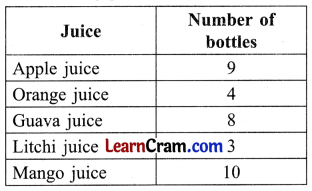Solution:Question 2.
Quantity of different varieties of sweets sold in a shop on one day is given below. Organise the information using pictures.Solution: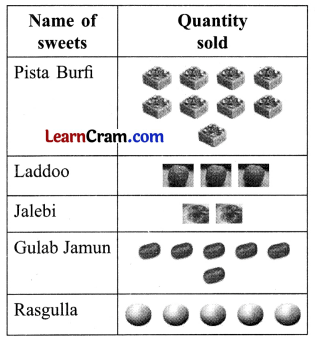Question 3.
Organise the information showing favourite colours of 30 children using pictures.Solution: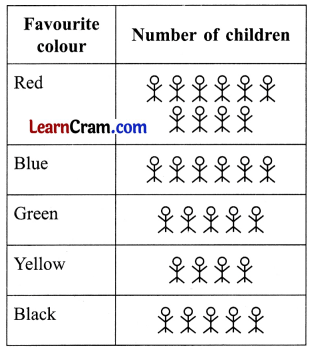Question 4.
Arrange the given information using tally marks.
(a) Number of books on different subjects in a library.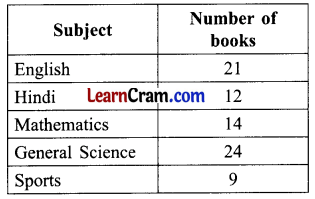Solution: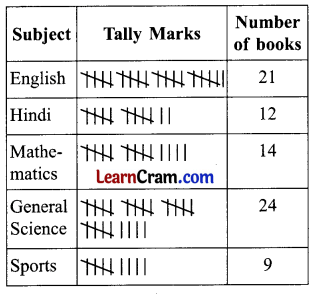(b) Number of different varieties of toffees in a packet.Solution: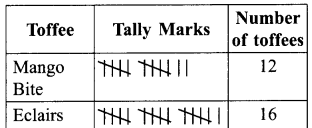(c) Number of different vehicles passing through a busy crossing in Delhi from 9 a.m. to 10 a.m.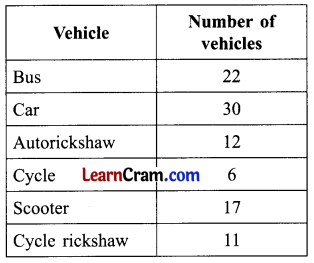Solution:Question 1.
Look at the table and tick (✓) the correct answer.
Number of students playing different games in the school.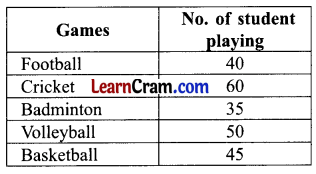(a) How much do children/students like Badminton?Solution:
35

(b) Most of the students like which game?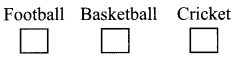Solution:
Cricket

(c) How many children like football?Solution:
40

(d) Which game is liked by the least number of students?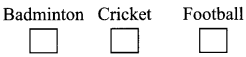Solution:Question 2.
A number of cars of a particular model produced by a factory in the year 2010 to 2014.(a) In which year the maximum number of cars were produced?
Solution:
2012

(b) In which year least number of cars were produced?
Solution:
2013

(c) In which years number of cars produced is same?
Solution:
2010, 2014

(d) Which year is considered best for the production of cars?
Solution:
2012

Question 3.
What is Tally Marks?
Solution:
In organising data lines are used to depict the object instead of drawing pictures. These lines are called Tally Mark.

Question 4.
Below is given pictograph showing the number of cars parked at a parking ground six days a week.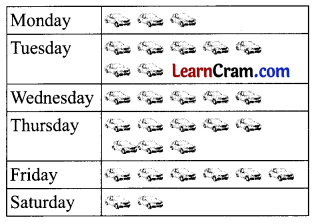(i) How many cars were parked on (a) Tuesday (b) Thursday
Solution:
(a) Tuesday – 7
(b) Thursday – 8

(ii) On which day the maximum number of cars were parked at the parking ground?
Solution:
Thursday

(iii) On which day the minimum number of cars were parked?
Solution:
Saturday

(iv) How many more cars were parked on Friday than on Wednesday?
Solution:
On Wednesday = 5 cars parked
On Friday = 6 cars parked
6 – 5 = 1
Extra car parked = 1

(v) What was the total number of cars parked in six days?
Solution:
Total cars parked = 31Question 5.
Arrange the given information using tally marks.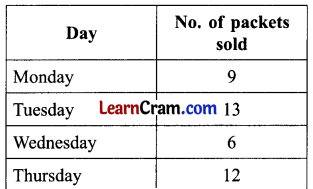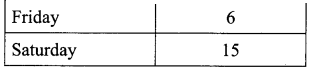Solution:Question 6.
Which is a better method, the pictorial method or the tally marks method in terms of time consumption?
Solution:
Tally marks

Question 7.
Organise the information showing favourite fruits of 30 children. Using tally marks.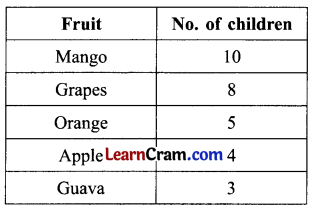Solution:Question 8.
Draw a pictograph for the following information and answer the following:(a) What is the total number of fruit trees in the orchard?
(b) How many orange trees are there?
(c) How many more apple trees are there than mango trees?
Solution:(a) 31
(b) 9
(c) 1Question 9.
Draw a pictograph for the following data.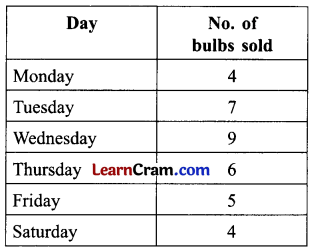Solution:Question 10.
Number of students using different soaps.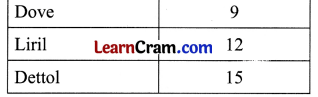Arrange the given information using tally marks.
Solution:DAV Class 5 Maths Chapter 17 Worksheet 2 Notes

Tally Marks
The following table shows the number of different types of trees in an orchard.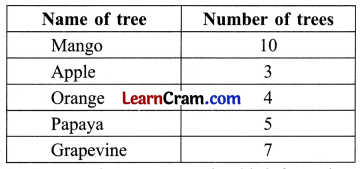Let us use pictures to organize this information.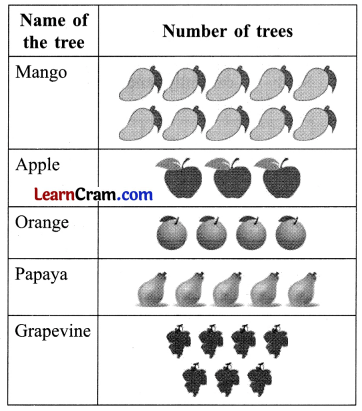Number of fruits shown is equal to number of fruit trees.
Instead of drawing pictures, we can also draw a line ( | ) where each line represents a tree. These lines are called Tally marks.
To make counting simple, the tally marks are made in groups of five. The fifth mark in each group is made as a cross.
Examples.